# Excel Sum formula examples - how to total a column, rows or only visible cells

The tutorial explains how to do sum in Excel by using the AutoSum feature, and how to make your own SUM formula to total a column, row or selected range. You will also learn how to sum only visible cells, calculate running total, sum across sheets, and find out why your Excel Sum formula is not working.

If you want a quick sum of certain cells in Excel, you can simply select those cells, and look at the status bar at the bottom right corner of your Excel window: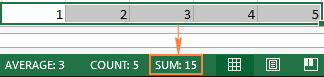For something more permanent, use the Excel SUM function. It is very simple and straightforward, so even if you are a beginner in Excel, you will hardly have any difficulty in understanding the following examples.

## How to sum in Excel using a simple arithmetic calculation

If you need a quick total of several cells, you can use Microsoft Excel as a mini calculator. Just utilize the plus sign operator (+) like in a normal arithmetic operation of addition. For example:

`=1+2+3`

or

`=A1+C1+D1`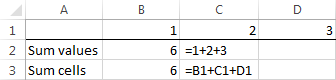However, if you need to sum a few dozen or a few hundred rows, referencing each cell in a formula does not sound like a good idea. In this case, you can use the Excel SUM function specially designed to add a specified set of numbers.

## How to use SUM function in Excel

Excel SUM is a math and trig function that adds values. The syntax of the SUM function is as follows:

SUM(number1, [number2] ,…)

The first argument is required, other numbers are optional, and you can supply up to 255 numbers in a single formula.

In your Excel SUM formula, each argument can be a positive or negative numeric value, range, or cell reference. For example:

`=SUM(A1:A100)`

`=SUM(A1, A2, A5)`

`=SUM(1,5,-2)`

The Excel SUM function is useful when you need to add up values from different ranges, or combine numeric values, cell references and ranges. For example:

`=SUM(A2:A4, A8:A9)`

`=SUM(A2:A6, A9, 10)`

The below screenshot shows these and a few more SUM formula examples: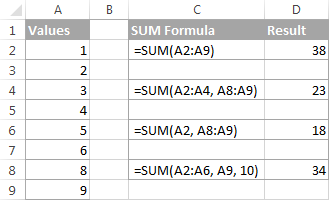In real-life worksheets, the Excel SUM function is often included in bigger formulas as part of more complex calculations.

For example, you can embed SUM in the value_if_true argument of the IF function to add numbers in columns B, C and D if all three cells in the same row contain values, and show a warning message if any of the cells is blank:

`=IF(AND(\$B2<"", \$C2<>"", \$D2<>""), SUM(\$B2:\$D2), "Value missing")`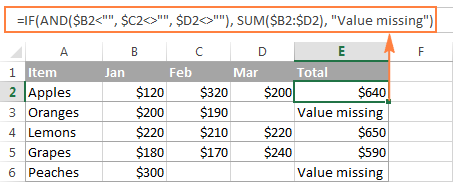And here's another example of using an advanced SUM formula in Excel: VLOOKUP and SUM formula to total all matching values.

## How to AutoSum in Excel

If you need to sum one range of numbers, whether a column, row or several adjacent columns or rows, you can let Microsoft Excel write an appropriate SUM formula for you.

Simply select a cell next to the numbers you want to add, click AutoSum on the Home tab, in the Editing group, press the Enter key, and you will have a Sum formula inserted automatically: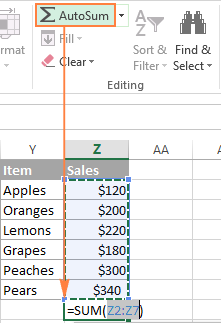As you can see in the following screenshot, Excel's AutoSum feature not only enters a Sum formula, but also selects the most likely range of cells that you'd want to total. Nine times out of ten, Excel gets the range right. If not, you can manually correct the range by simply dragging the cursor through the cells to sum, and then hit the Enter key.

Tip. A faster way to do AutoSum in Excel is to use the Sum shortcut Alt + =. Just hold the Alt key, press the Equal Sign key, and then hit Enter to complete an automatically inserted Sum formula.

Apart from calculating total, you can use AutoSum to automatically enter AVERAGE, COUNT, MAX, or MIN functions. For more information, please check out the Excel AutoSum tutorial.

## How to sum a column in Excel

To sum numbers in a specific column, you can use either the Excel SUM function or AutoSum feature.

For example, to sum values in column B, say in cells B2 to B8, enter the following Excel SUM formula:

`=SUM(B2:B8)`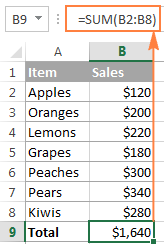#### Total an entire column with indefinite number of rows

If a column you want to sum has a variable number of rows (i.e. new cells can be added and existing ones can be deleted at any time), you can sum the entire column by supplying a column reference, without specifying a lower or upper bound. For example:

`=SUM(B:B)`

Important note! In no case you should put your 'Sum of a column' formula in the column you want to total because this would create a circular cell reference (i.e. an endless recursive summation), and your Sum formula would return 0.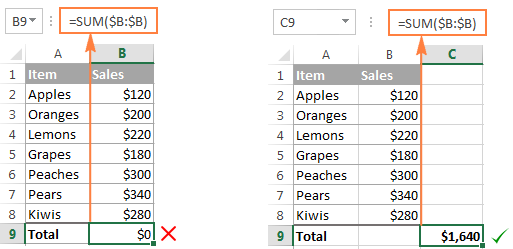#### Sum column except header or excluding a few first rows

Usually, supplying a column reference to the Excel Sum formula totals the entire column ignoring the header, as demonstrated in the above screenshot. But in some cases, the header of the column you want to total can actually have a number in it. Or, you may want to exclude the first few rows with numbers that are not relevant to the data you want to sum.

Regrettably, Microsoft Excel does not accept a mixed SUM formula with an explicit lower bound but without an upper bound like =SUM(B2:B), which works fine in Google Sheets. To exclude the first few rows from summation, you can use one of the following workarounds.

• Sum the entire column and then subtract the cells you don't want to include in the total (cells B1 to B3 in this example):

`=SUM(B:B)-SUM(B1:B3)`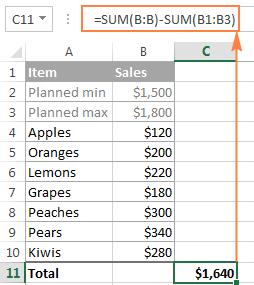• Remembering the worksheet size limits, you can specify the upper bound of your Excel SUM formula based on the maximum number of rows in your Excel version.

For example, to sum column B without the header (i.e. excluding cell B1), you can use the following formulas:

• In Excel 2007, Excel 2010, Excel 2013, and Excel 2016:
`=SUM(B2:B1048576)`
• In Excel 2003 and lower:
`=SUM(B2:B655366)`

## How to sum rows in Excel

Similarly to totaling a column, you can sum a row in Excel by using the SUM function, or have AutoSum to insert the formula for you.

For example, to add values in cells B2 to D2, use the following formula:

`=SUM(B2:D2)`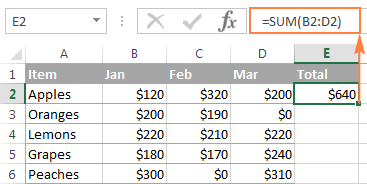#### How to sum multiple rows in Excel

To add values in each row individually, just drag down your Sum formula. The key point is to use relative (without \$) or mixed cell references (where the \$ sign fixes only the columns). For example:

`=SUM(\$B2:\$D2)`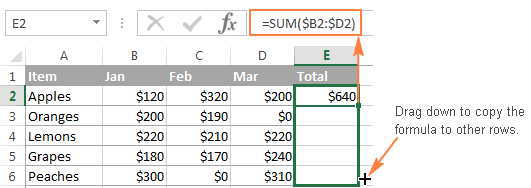To total the values in a range containing several rows, simply specify the desired range in the Sum formula. For example:

`=SUM(B2:D6)` - sums values in rows 2 to 6.

`=SUM(B2:D3, B5:D6)` - sums values in rows 2, 3, 5 and 6.

#### How to sum a whole row

To sum the entire row with an indefinite number of columns, supply a whole-row reference to your Excel Sum formula, e.g.:

`=SUM(2:2)`

Please remember that you shouldn't enter that 'Sum of a row' formula in any cell of the same row to avoid creating a circular reference because this would result in a wrong calculation, if any: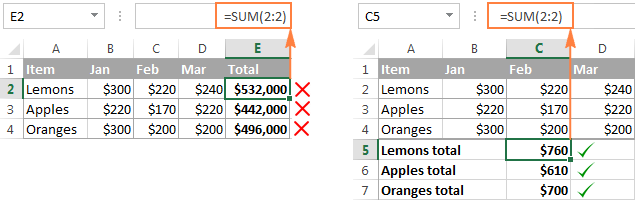To sum rows excluding a certain column(s), total the entire row and then subtract irrelevant columns. For example, to sum row 2 except the first 2 columns, use the following formula:

`=SUM(2:2)-SUM(A2:B2)`

## Use Excel Total Row to sum data in a table

If your data is organized in an Excel table, you can benefit from the special Total Row feature that can quickly sum the data in your table and display totals in the last row.

A big advantage of using Excel tables is that they auto-expand to include new rows, so any new data you input in a table will be included in your formulas automatically. If can learn about other benefits of Excel tables in this article: 10 most useful features of Excel tables.

To convert an ordinary range of cells into a table, select it and press Ctrl + T shortcut (or click Table on the Insert tab).

#### How to add a total row in Excel tables

Once your data is arranged in a table, you can insert a total row in this way:

1. Click anywhere in the table to display the Table Tools with the Design tab.
2. On the Design tab, in the Table Style Options group, select the Total Row box: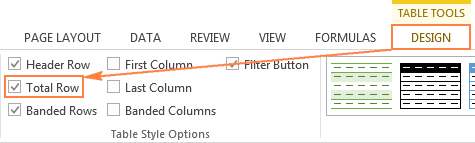Another way to add a total row in Excel is to right click any cell within the table, and then click Table > Totals Row.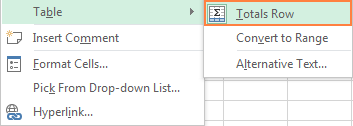#### How to total data in your table

When the total row appears at the end of the table, Excel does its best to determine how you would like to calculate data in the table.

In my sample table, the values in column D (rightmost column) are added automatically and the sum is displayed in the Total Row: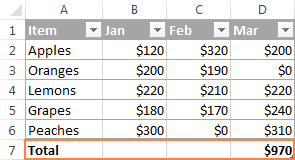To total values in other columns, simply select a corresponding cell in the total row, click the drop-down list arrow, and select Sum: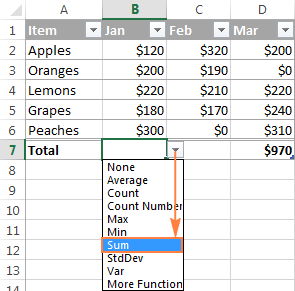If you want to perform some other calculation, select the corresponding function from the drop-down list such as Average, Count, Max, Min, etc.

If the total row automatically displays a total for a column that doesn't need one, open the dropdown list for that column and select None.

Note. When using the Excel Total Row feature to sum a column, Excel totals values only in visible rows by inserting the SUBTOTAL function with the first argument set to 109. You will find the detailed explanation of this function in the next section.

If you want to sum data both in visible and invisible rows, do not add the total row, and use a normal SUM function instead: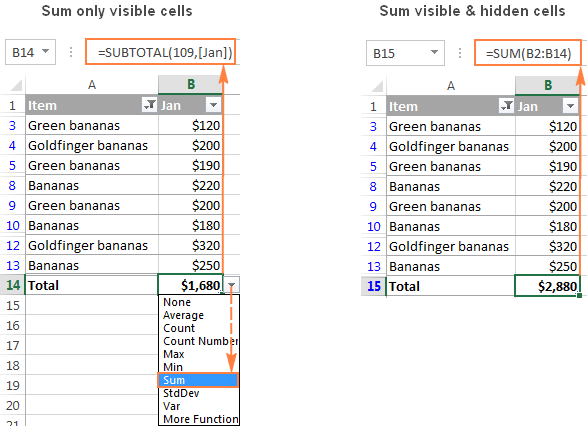## How to sum only filtered (visible) cells in Excel

Sometimes, for more effective date analysis, you may need to filter or hide some data in your worksheet. A usual Sum formula won't work in this case because the Excel SUM function adds all values in the specified range including the hidden (filtered out) rows.

If you want to sum only visible cells in a filtered list, the fastest way is to organize your data in an Excel table, and then turn on the Excel Total Row feature. As demonstrated in the previous example, selecting Sum in a table's total row inserts the SUBTOTAL function that ignores hidden cells.

Another way to sum filtered cells in Excel is to apply an AutoFilter to your data manually by clicking the Filter button on the Data tab. And then, write a Subtotal formula yourself.

The SUBTOTAL function has the following syntax:

SUBTOTAL(function_num, ref1, [ref2],…)

Where:

• Function_num- a number from 1 to 11 or from 101 to 111 that specifies which function to use for the subtotal.

You can find the full list of functions on support.office.com. For now, we are interested only in the SUM function, which is defined by numbers 9 and 109. Both numbers exclude filtered-out rows. The difference is that 9 includes cells hidden manually (i.e. right-click > Hide), while 109 excludes them.

So, if you are looking to sum only visible cells, regardless of how exactly irrelevant rows were hidden, then use 109 in the first argument of your Subtotal formula.

• Ref1, Ref2, … - cells or ranges that you want to subtotal. The first Ref argument is required, others (up to 254) are optional.

In this example, let's sum visible cells in range B2:B14 by using the following formula:

`=SUBTOTAL(109, B2:B14)`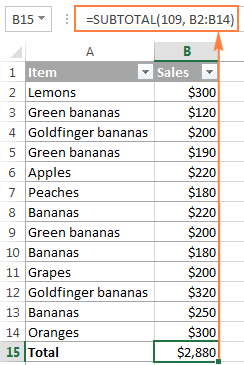And now, let's filter only 'Banana' rows and make sure that our Subtotal formula sums only visible cells: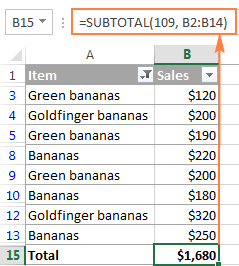Tip. You can have Excel's AutoSum feature to insert the Subtotal formula for you automatically. Just organize your data in table (Ctrl + T) or filter the data the way you want by clicking the Filter button. After that, select the cell immediately below the column you want to total, and click the AutoSum button on the ribbon. A SUBTOTAL formula will be inserted, summing only the visible cells in the column.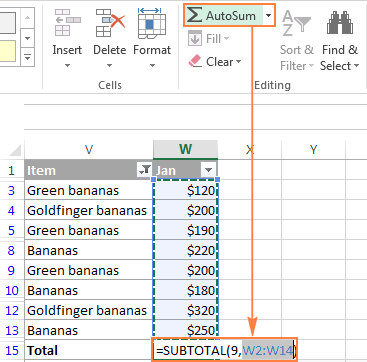## How to do a running total (cumulative sum) in Excel

To calculate a running total in Excel, you write a usual SUM formula with a clever use of absolute and relative cells references.

For example, to display the cumulative sum of numbers in column B, enter the following formula in C2, and then copy it down to other cells:

`=SUM(\$B\$2:B2)`

The relative reference B2 will change automatically based on the relative position of the row in which the formula is copied: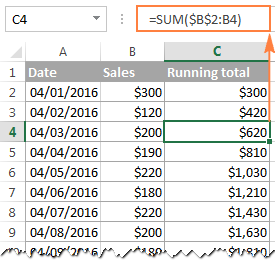You can find the detailed explanation of this basic Cumulative Sum formula and tips on how to improve it in this tutorial: How to calculate running total in Excel.

## How to sum across sheets

If you have several worksheets with the same layout and the same data type, you can add the values in the same cell or in the same range of cells in different sheets with a single SUM formula.

A so-called 3-D reference is what does the trick:

`=SUM(Jan:Apr!B6)`

Or

`=SUM(Jan:Apr!B2:B5)`

The first formula adds values in cell B6, while the second formula sums the range B2:B5 in all worksheets located between the two boundary sheets that you specify (Jan and Apr in this example):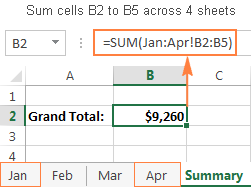You can find more information about a 3-d reference and the detailed steps to create such formulas in this tutorial: How to create a 3-D reference to calculate multiple sheets.

## Excel conditional sum

If your task requires adding only those cells that meet a certain condition or a few conditions, you can use the SUMIF or SUMIFS function, respectively.

For example, the following SUMIF formula adds only those amounts in column B that have "Completed" status in column C:

`=SUMIF(C:C,"completed",B:B )`To calculate a conditional sum with multiple criteria, use the SUMIFS function. In the above example, to get the total of "Completed" orders with the amount over \$200, use the following SUMIFS formula:

`=SUMIFS(B:B,C:C,"completed",B:B, ">200" )`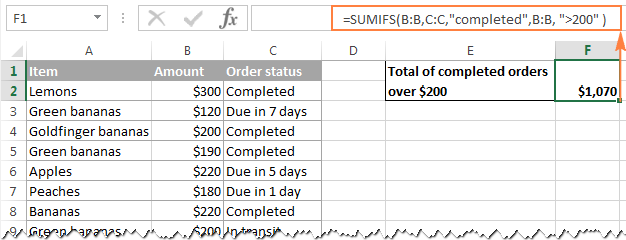You can find the detailed explanation of the SUMIF and SUMIFS syntax and plenty more formula examples in these tutorials:

Note. The Conditional Sum functions are available in Excel versions beginning with Excel 2003 (more precisely, SUMIF was introduced in Excel 2003, while SUMIFS only in Excel 2007). If someone still uses an earlier Excel version, you'd need to make an array SUM formula as demonstrated in Using Excel SUM in array formulas to conditionally sum cells.

## Excel SUM not working - reasons and solutions

Are you trying to add a few values or total a column in your Excel sheet, but a simple SUM formula doesn't compute? Well, if the Excel SUM function is not working, it's most likely because of the following reasons.

#### 1. #Name error appears instead of the expected result

It's the easiest error to fix. In 99 out of 100 cases, the #Name error indicates that the SUM function is misspelled.

#### 2. Some numbers are not added

Another common reason for a Sum formula (or Excel AutoSum) not working are numbers formatted as text values. At first sight, they look like normal numbers, but Microsoft Excel perceives them as text strings and leaves them out of calculations.

One of the visual indicators of text-numbers are the default left alignment and green triangles in top-left corner of the cells, like in the right-hand sheet in the below screenshot: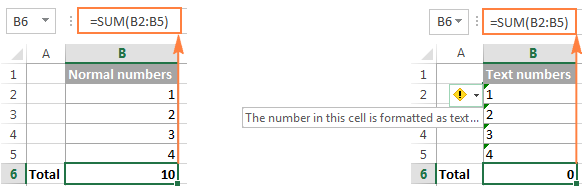To fix this, select all problematic cells, click the warning sign, and then click Convert to Number.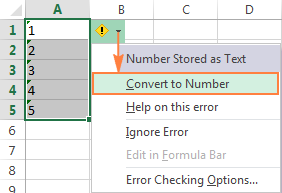If against all expectations that does not work, try other solutions described in: How to fix numbers formatted as text.

#### 3. Excel SUM function returns 0

Apart from numbers formatted as text, a circular reference is a common source of problem in Sum formulas, especially when you are trying to total a column in Excel. So, if your numbers are formatted as numbers, but your Excel Sum formula still returns zero, trace and fix the circular references in your sheet (Formula tab > Error Checking > Circular Reference). For the detailed instructions, please see How to find a circular reference in Excel.

#### 4. Excel Sum formula returns a higher number than expected

If against all expectations your Sum formula returns a bigger number than it should, remember that the SUM function in Excel adds both visible and invisible (hidden) cells. In this case, use the Subtotal function instead, as demonstrated in How to sum only visible cells in Excel.

#### 5. Excel SUM formula not updating

When a SUM formula in Excel continues to show the old total even after you've updated the values in the dependent cells, most likely Calculation Mode is set to Manual. To fix this, go to the Formulas tab, click the dropdown arrow next to Calculate Options, and click Automatic.

Well, these are the most common reasons for SUM not working in Excel. If none of the above is your case, check out other possible reasons and solutions: Excel formulas not working, not updating, not calculating.

This is how you use a SUM function in Excel. If you want to have a closer look at the formula examples discussed in this tutorial, you are welcome to download a sample Excel SUM workbook. I thank you for reading and hope to see you on our blog next week.

### 51 responses to "Excel Sum formula examples - how to total a column, rows or only visible cells"

1.Amaral says:

thanks for the tip on How to sum only filtered!!!

2.Sahil says:

I have a data table that is updated daily, for example, a new column is added daily, I want to find the difference between the last updated column & first column. But, don’t want to rewrite formula each time.
Can you help me...

3.Shirine says:

I'm a new user I have a units and and unit cost say I have 50 units and each are 1.99 how do I get home he sum.I would really appreciate it.

4.prashant kaushik says:

ms office is the heart of computer programing

5.bharath says:

6.Tina Law says:

What is the formula to sum up a total billing per Customer# by Customer name. I also need a formula of average billing and the highest billing per Customer.

Example:
Column A B C D E F
Customer# Customer Name Bill Amt Total Pd Amt Avg Bill Total Amt YTD
1 A \$125 \$125
1 A \$0 \$0
2 B \$85 \$85
2 B \$225 \$225
3 C \$35 \$35
3 C \$67 \$67
4 D \$324 \$324
4 D \$455 \$455
4 D \$124 \$124
4 D \$0 \$0

I need the SUMIF formula if applicable. Thank you for your help.

Tina

7.Tina Law says:

What is the formula to sum up a total billing per Customer# by Customer name. I also need a formula of average billing and the highest billing per Customer.

Example:
Column A B C D E F
Customer# Customer Name Bill Amt Total Pd Amt Avg Bill HighestBill YTD
1 A \$125 \$125
1 A \$0 \$0
2 B \$85 \$85
2 B \$225 \$225
3 C \$35 \$35
3 C \$67 \$67
4 D \$324 \$324
4 D \$455 \$455
4 D \$124 \$124
4 D \$0 \$0

I need the SUMIF formula if applicable. Thank you for your help.

This formula is good but can I replace row number for customer name? =IF(AND(\$B2<"", \$C2"", \$D2""), SUM(\$B2:\$D2), "Value missing")

Tina

8.Gaurav says:

How add if table like 24kg
25 kg

9.jackie says:

How to sum a whole row

To sum the entire row with an indefinite number of columns, supply a whole-row reference to your Excel Sum formula, e.g.:

=SUM(2:2)

Please remember that you shouldn't enter that 'Sum of a row' formula in any cell of the same row to avoid creating a circular reference because this would result in a wrong calculation, if any:
mam please elaborate this with xls file i am not able to understand it

10.KASAUDHAN says:

Dear Sir,

actually i have approximately 25 row in that i want to sum like this way
1row +3rd row + 5th row + 7th row ...... if there is any formula please provide me

11.Thomas Connor says:

lots of good stuff herein - thanks!

But, I want to make bold the "sum" product of auto-summing in the bottom bar of the Excel screen (where "sum" is accompanied by "average" and "count").

Can this be accomplished?

I'm 85 and even with glasses the sum product is not very visible. Microsoft should change this and make it bold. All octogenarians would give a shout-out if they did.

Tom

12.Rizwan says:

I want to know i have excel sheet i want to fix the SUM formula after filter the sheet in some selected row actually i have tried after filter but which cell is hide that cell Qty also adding in total please check tell me if you have any formula.

•siva says:

=Subtotal(9,c2:c6)

inplace of c2:c6 substitute the column and row details as per your worksheet

•jayesh says:

hello, I have another doubt in which i was able to add filted numbers but when i open the unfiltered sheet it goes back to sum of all the numbers. please help.
i filtered the items to make a total of different items but when i change the filter to "all" it changes to sum of all the numbers in the range.

13.Candi says:

I want to run an inventory sheet where I can take from a beginning number or add to that same number and have it give me a quantity on hand. Example. I have 20 of something and tomorrow I remove 4 of them so I need a box to calculate that I now have 16 but the next day I add 10 back into the qty- I now want that box to show I have 26.

14.Barbara says:

I have a budget worksheet by month. Totaled income/expense at the end of each month. Jan=column B etc....but when adding income/expense to Feb (column C) is adds or remove from my January total.

My formula for January is =SUM(B62-B49)so why is adding to column C effecting this?

Thank you

15.Raja says:

i have small doubt in excel pl solve this. details given below
i have on table some rows and columns
sl.no. amend no. scope qty amt
1 1 1234 1500 1000.00
2 2 1234 50 800.00
3 1 324 100 200.00
4 1 545 10 50.00
6 2 324 87 65.00
7 3 324 54 85.00

Now i want like every scope highest amend no details.
pl help me

16.Paul says:

I want to total all all rows in Column A minus Column row1,2,3... sum into Column C row 1,2,3... need a formula please

17.Paul says:

I want to total all all rows in Column A minus Column B row1,2,3... sum into Column C row 1,2,3... need a formula please

•Natalia Sharashova (Ablebits.com Team) says:

Hello, Paul,
try using the following:
=SUM(A:A)-SUM(B1:B3)+SUM(C1:C3)

18.samuel sushant says:

it is very well...i cleared all my doubts...but how i can iget ablebits in my windows 10 computer

19.shivam says:

Ram 2000
Raj 400
Pop 221
Arun 339
sohan 3382

I want to calculate the total of all.. but skip "pop" and "ram" automatically....
Eg:
Ram 2000
Raj 400
Pop 221
Arun 339
sohan 3382

the total of this will be "6342." but it automatically skip pop & ram & give answer "7721."

20.JAWAHAR says:

how to calculate the sum of the following?
(G7X5)+(H7X4)+(I7X3)+(J7X2)+(K7X1)

21.JAWAHAR says:

how to compute (G7x5)+(H7x4)in a horizontal row

22.kunal kumar says:

how to calculate total without sum function or + operator

23.Vaishali Mhaske says:

Please solved this example : -

Analyze Data Actual Default
A 486 53
B 150
C 10
Total

24.Upendra says:

Hello everyone,Challanging question for you all (Please understand the issue carefully before answering hapazardly)
C1:C10 (weight gain)= 1,2,3,4,5,6,7,8,9,10
A1:A10 Age of child in month) = 11,20,23,40,55,60,32,80,90,95,160
Now I make class interval in B1:B10 as (0-1)(2-6)(7-12)(13-24)(25-60)(61-168) for A1:A10
I did: sum age of child meeting (0-1) in B1 which is always showing 0 gram weight increase, for those child who doesnot exist (mistake calculation), want to display empty cell for such case and sum only of there is value in column J. Formulas working fine for the existing child.

I couldnot localize how to calculate for those rows that donot exist in A and display empty cells in B.
Again, for reminder: excel is showing 0 gram increase for those child that doesnot exist in my excel sheet.
I tried 100s of formulas but non of them worked.
Please suggest same formula for all calculating in all class intervals as mentioned above

25.Upendra says:

Hello everyone,Challanging question for me (Please understand the issue carefully before answering hapazardly)
C1:C10 (weight gain)= 1,2,3,4,5,6,7,8,9,10
A1:A10 Age of child in month) = 11,20,23,40,55,60,32,80,90,95,160
Now I make class interval in B1:B6 as (0-1)(2-6)(7-12)(13-24)(25-60)(61-168) for A1:A10
I did: sum age of child meeting (0-1) in B1 which is always showing 0 gram weight increase, for those child who doesnot exist (mistake calculation), want to display empty cell for such case and sum only of there is value in column B. Formulas working fine for the existing child.
I couldnot localize how to calculate for those rows that donot exist in A and display empty cells in B.
Again, for reminder: excel is showing 0 gram increase for those child that doesnot exist in my excel sheet.
I tried 100s of formulas but non of them worked.
Please suggest same formula for all calculating in all class intervals as mentioned above

26.shelly says:

I would appreciate if someone could help. I have an excel document that is exported from simply. It has a column with subtotals (=SUBTOTAL(9,H12:H17))for each property and I would like to total the subtotals without having to do in manually.(c5+c17+c27) Thank you in advance

27.Rey says:

I am trying to sum columns and if the total is 40or more, change the total to 40 which is the set up maximum. And if the total is less than 40, then just show the correct total. Can somebody help me with the formula? Thank you.

•Gennady Terekhov (Ablebits.com Team) says:

Hello, Rey,

=IF(SUM(A:A)>40,40,SUM(A:A))

28.J says:

My problem is viewing:
If I enter amounts with decimals or cents I am not able to view the whole total (the amounts to the right of the decimal point). How do I extend the area to view the entire total?

29.Raju Reddy says:

How to find the sum of formula cells only ?

30.Bikach Chandra Paul says:

I have a problem to sum only the digits which cells are formatted by kg, like

5 kgs
4 yds
3 kgs
2 kgs
7 yds
_______________

I would like get sum result only with the kgs, above result should be 10 kgs.
Could you pls advise how to solve it.

•Doug says:

It can be done pretty simply if you split the text from the numbers like this:
Where the numbers are in A29:A33 and the text is in B29:B33 the formula is:
=SUMIF(B29:B33,"*kgs*",A29:A33)

31.MARK says:

Hi,
How do I sum visable cells only(filtered)
VISIBLE CELLS POPULATE A ROW

COLUMN (FILTERED)
C G H K L N

ROW...... 6 3 5 9 2 4 = SUM ?

Thank you

32.Abdul says:

Too much thanks, It's very useful.

33.Sara says:

I have to find the cgpa of my class result...i have done summing up amd grading in excel sheet...but cant find out the formula

34.Johanna says:

the auto sum for visible data (not hidden) only instructions do not work

35.arash says:

Thanks. It helped me a lot.

36.ssa says:

today i enter the value in column B5 and the total of column B5=c55, next day i enter the value in column b5, then it must added automatically in column C5 automatically

37.henry says:

In a file, i maintain 2 sheet summary and detail. Detail sheet is datewise and summary sheet is tank no wise, so i did filter in detail sheet and in summary sheet i used formula of subtotal(9,(range)) for calculating total amount against particular tanker. But when i remove filter, total of summary sheet gets changed. So please let me know which formula I have to used.
Thanks.

38.pav says:

Question 1: Can someone help me understand how this formula works
A1:1
A2:2
A2:3
=Sum(A1:A3-1)= 2 ## Need help with this Question?
Question 2:
A1 B1 C1
=Sum(A1:C1)-Sum(A1-1:C1-1) ## this Formula is not working, any comments?

39.Sudip C. Pal says:

D/Sir
To find the Total I am using the formula subtotal(109,M10:U10) but the result is adding the hidden columns also.Any solution to this problem?
Thanks
Sudip C. Pal

40.namere allanz says:

41.namere allanz says:

define for me the following terms used in spreadsheet
Function
An operator

42.hakeem says:

Please help me,To find the automatically sum amount of particular raw if i colored raw.for example i prepared chart of receivable amount.when i received money i just shide the color on who gave money.i want to know how to make a formula to avoid the colored raw.please help me

43.ArmoTxa says:

Thank you 4 gr8 infos

44.Adrian Cargill says:

Thanks for the comprehensive answer to how to sum only visible cells. Very helpful.

45.Mirza Faisal Hussain says:

Thanks, it works

46.Md. Abdul Mazed says:

My requirement is auto sl. number under filtering data . if any sl. no. of row is invisible which to be auto numbered with next visible raw . Is it possible to formulated my requirement. As example if i invisible the raw no. 2. by Filtered data.

Sl. No. Vendor Code Company Code Vendor Name City

1 10001784 2100 Mt Bashundhara Lpg-3 Dhaka
2 10003438 2100 MT. Bashundhara LPG 6 Umme Kulsum Road,Bashundhara R/A
3 10003484 2100 M. T. Bashundhara LPG-01 Pangaon, Konda, South Keranigonj,Dh
4 50003794 2100 MT. Bashundhara LPG 2 Dhaka
5 60000018 2100 M. T. BASHUNDHARA LPG-04 Dhaka
6 60000019 2100 M. T. Bashundhara LPG-05 Dhaka